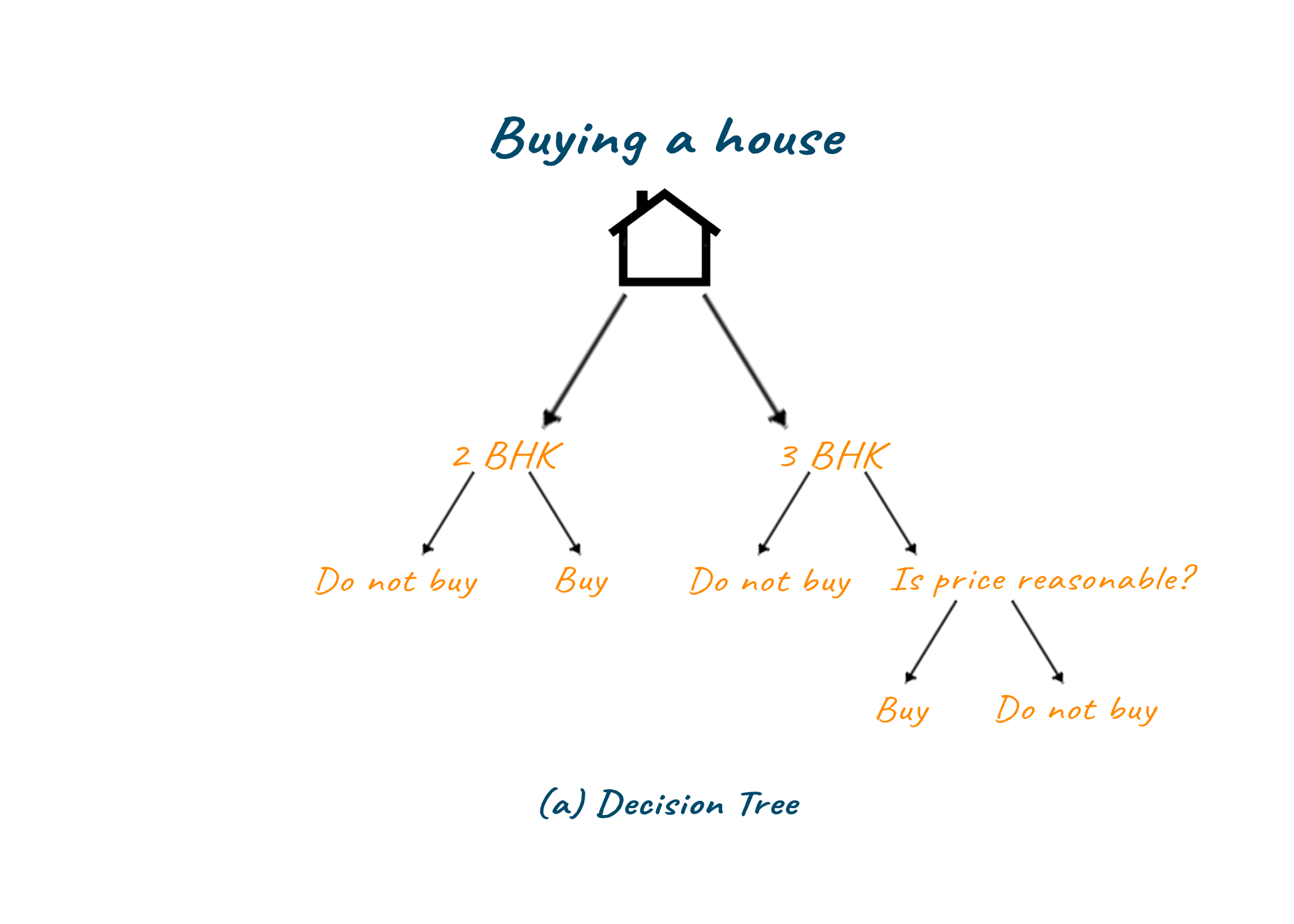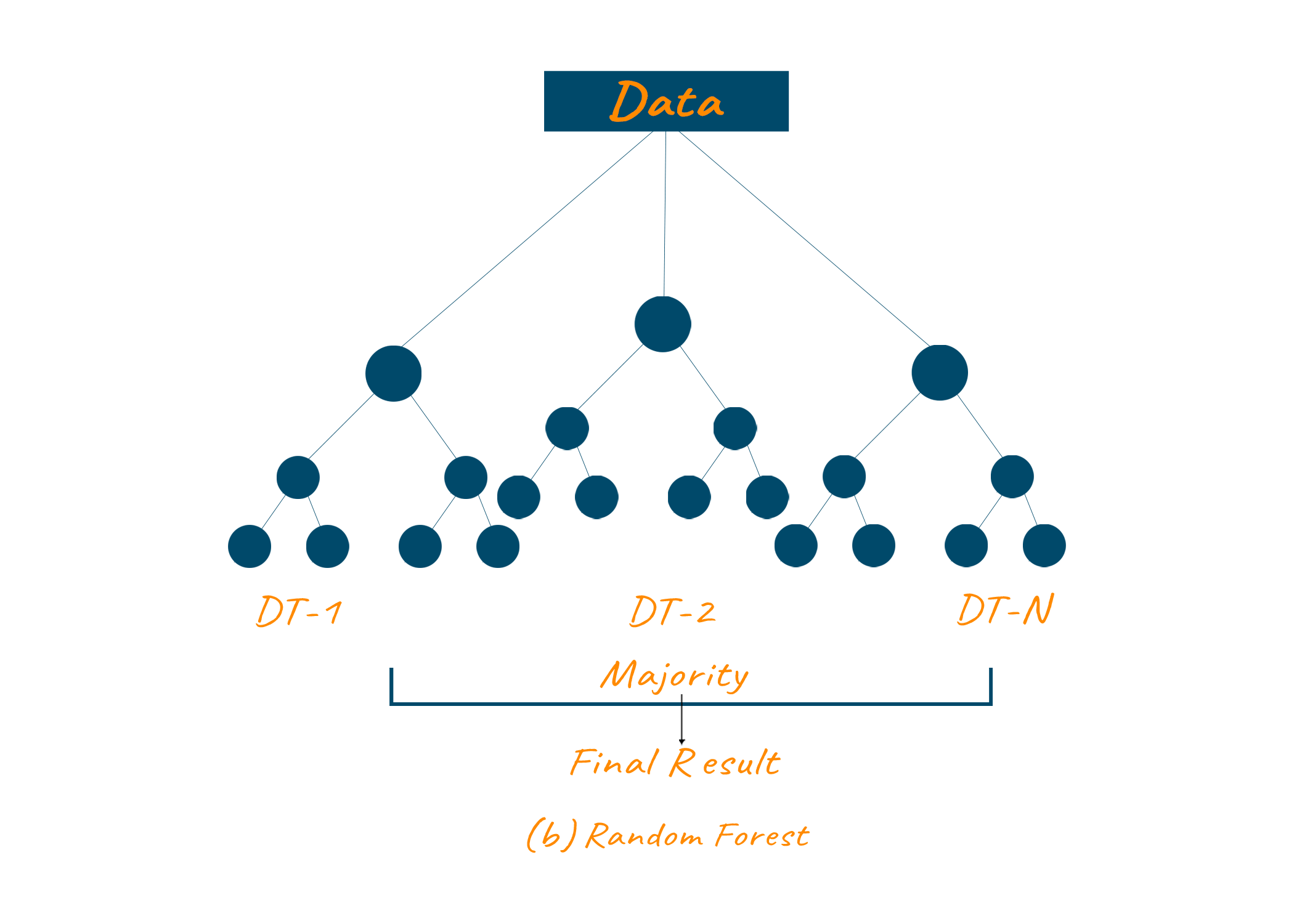# Decision Tree vs Random Forest (10 Differences)

As digital data is exponentially increasing day by day, several algorithms came to deal with that data. Choosing the right machine learning algorithm for a particular problem is the most important aspect to build a better model. The efficiency of the algorithm determines the execution speed of the mode. Basically, algorithms are developed by mathematical concepts and problems. It can be used to handle and analyze massive amounts of data. In this article let us compare the two important algorithms decision tree and random forest with their pros and cons.

## What is a Decision tree?

By the name, we can know that this algorithm is based on the structure of the tree. A decision tree is one of the important supervised learning algorithms for dealing with classification and regression problems. The structure of the decision tree algorithm is basically compared to an actual tree. The branches in the tree represent the decisions while the leaf represents the result of the decision. It can handle both categorical and continuous data. To traverse the nodes in the decision tree, recursion is mainly used.

### Example

Let us take an example to understand the working of decision tree algorithms in a better way. If you are planning to buy a house, first you are deciding whether you need a 2BHK house or a 3BHK house according to your requirements. Then after making the decision you will check the price for the corresponding house and after that, you will make your final decision on whether you want to buy that house or not. The working of the decision tree is similar to this. Let us look at this simple and effective decision-making process as a diagram.• The major advantage of the decision tree is that the model is easy to interpret.
• The larger the data the better will be the results
• Less data cleaning is required

• The rate of deflection is high in the case of decision trees.
• A slight change in data may cause a significant change in the result.
• It is prone to errors like overfitting, bias, and variance

## What is Random Forest?

Random forest is a supervised learning algorithm in which a group of decisions is made and the result is finalized based on the majority. Hence we can say it is a collection of decision trees. As the name suggests forest may contain a group of trees the algorithm contains a collection of decision trees. Since multiple decision trees are grouped to build the random forest it is more complicated.

### Example

Let us take an example to understand the working of a random forest algorithm in a better way. If you are planning to buy a new house and you have multiple options like A, B, C, and so on. So in this case we have to build a decision tree for each case and group them together to find the best option. We have to build separate decision trees for each option A, B, C, etc., in the same way, we have built a single decision tree. After that output from each tree is analyzed with a predictive system to find the desired result.• The random forest algorithm is highly accurate and powerful.
• The algorithm adapts quickly to the dataset
• It can handle several features at once

• The major drawback of the random forest model is its complicated structure due to the grouping of multiple decision trees.
• It cannot be used for linear patterns of data.
• It is worse for handling data with high dimension

## Difference Between Decision Tree and Random Forest

 Comparison basis Decision Tree Random Forest Speed It is fast It is slow Interpretation It is easy to interpret It is quite complex to interpret Time Takes less time Takes more time Linear problems It is best to build solutions for linear patterns of data It cannot handle data with linear patterns Overfitting There is a possibility of overfitting of data There is a reduced risk of overfitting, because of the multiple trees Computation It has less computation It has more computation Visualization Visualization is quite simple Visualization is quite complex Outliers Highly prone to being affected by outliers Much less likely to be affected by outliers Implementation Rapid model building as it fits the dataset quickly. Slow to build the model depending on the size of the dataset Accuracy It gives less accurate results It gives more accurate results

## When to Use Decision Tree vs Random Forest?

The choice to make between the decision tree and the random forest is crucial and completely depends on the problem type. If you are building a quick model where you don't bother about the accuracy much then you can go for a decision tree. Since it can be built and easy process. But if you want to build a better predictive system with more accuracy and time is not a constraint then you can go for the random forest. In which you can build a better model with a slow process. As the decision tree is fast it operates easily on large datasets whereas the random forest needs rigorous training for large datasets.

## Conclusion

As we have discussed, each algorithm has its own purpose with pros and cons. We have to analyze the problem and then have to choose the perfect algorithm. By this article, you might have understood the major differences between decision tree and random forest algorithms. So, try to start the implementation of these algorithms to get much better exposure to these topics.

### FavTutor - 24x7 Live Coding Help from Expert Tutors!